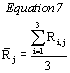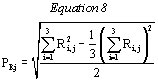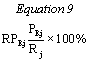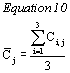# Title 40

## SECTION 53.34

### 53.34 Test procedure for methods for PM10 and Class I methods for PM 2.5.

§ 53.34 Test procedure for methods for PM10 and Class I methods for PM 2.5.

(a) Comparability. Comparability is shown for PM 10 methods and for Class I methods for PM 2.5 when the relationship between:

(1) Measurements made by a candidate method, and

(2) Measurements made by a corresponding reference method on simultaneously collected samples (or the same sample, if applicable) at each of one or more test sites (as required) is such that the linear regression parameters (slope, intercept, and correlation coefficient) describing the relationship meet the requirements specified in table C-4 of this subpart.

(b) Methods for PM10. Test measurements must be made, or derived from particulate samples collected, at not less than two test sites, each of which must be located in a geographical area characterized by ambient particulate matter that is significantly different in nature and composition from that at the other test site(s). Augmentation of pollutant concentrations is not permitted, hence appropriate test sites must be selected to provide the minimum number of test PM 10 concentrations in the ranges specified in table C-4 of this subpart. The tests at the two sites may be conducted in different calendar seasons, if appropriate, to provide PM 10 concentrations in the specified ranges.

(c) PM10 methods employing the same sampling procedure as the reference method but a different analytical method. Candidate methods for PM 10 which employ a sampler and sample collection procedure that are identical to the sampler and sample collection procedure specified in the reference method, but use a different analytical procedure, may be tested by analyzing common samples. The common samples shall be collected according to the sample collection procedure specified by the reference method and shall be analyzed in accordance with the analytical procedures of both the candidate method and the reference method.

(d) Methods for PM2.5. Augmentation of pollutant concentrations is not permitted, hence appropriate test sites must be selected to provide the minimum number of test measurement sets to meet the requirements for PM 2.5 concentrations in the ranges specified in table C-4 of this subpart. Only one test site is required, and the site need only meet the PM 2.5 ambient concentration levels required by table C-4 of this subpart and the requirements of § 53.30(b) of this subpart. A total of 10 valid measurement sets is required.

(e) Collocated measurements. (1) Set up three reference method samplers collocated with three candidate method samplers or analyzers at each of the number of test sites specified in table C-4 of this subpart.

(2) The ambient air intake points of all the candidate and reference method collocated samplers or analyzers shall be positioned at the same height above the ground level, and between 2 meters (1 meter for samplers or analyzers with flow rates less than 200 L/min) and 4 meters apart. The samplers shall be oriented in a manner that will minimize spatial and wind directional effects on sample collection.

(3) At each site, obtain as many sets of simultaneous PM 10 or PM 2.5 measurements as necessary (see table C-4 of this subpart), each set consisting of three reference method and three candidate method measurements, all obtained simultaneously.

(4) Candidate PM 10 method measurements shall be nominal 24-hour (±1 hour) integrated measurements or shall be averaged to obtain the mean concentration for a nominal 24-hour period. PM 2.5 measurements may be either nominal 24-or 48-hour integrated measurements. All collocated measurements in a measurement set must cover the same nominal 24-or 48-hour time period.

(5) For samplers, retrieve the samples promptly after sample collection and analyze each sample according to the reference method or candidate method, as appropriate, and determine the PM 10 or PM 2.5 concentration in µg/m 3. If the conditions of paragraph (c) of this section apply, collect sample sets only with the three reference method samplers. Guidance for quality assurance procedures for PM 2.5 methods is found in “Quality Assurance Document 2.12” (reference (2) in appendix A to this subpart).

(f) Sequential samplers. For sequential samplers, the sampler shall be configured for the maximum number of sequential samples and shall be set for automatic collection of all samples sequentially such that the test samples are collected equally, to the extent possible, among all available sequential channels or utilizing the full available sequential capability.

(g) Calculation of reference method averages and precisions. (1) For each of the measurement sets, calculate the average PM 10 or PM 2.5 concentration obtained with the reference method samplers, using equation 7 of this section:Where: R = The concentration measurements from the reference methods; i = The sampler number; and j = The measurement set number.

(2) For each of the measurement sets, calculate the precision of the reference method PM 10 or PM 2.5 measurements as the standard deviation, PRj, using equation 8 of this section:(3) For each measurement set, also calculate the precision of the reference method PM 10 or PM 2.5 measurements as the relative standard deviation, RPRj, in percent, using equation 9 of this section:(h) Acceptability of measurement sets. Each measurement set is acceptable and valid only if the three reference method measurements and the three candidate method measurements are obtained and are valid, R j falls within the acceptable concentration range specified in table C-4 of this subpart, and either PRj or RPRj is within the corresponding limit for reference method precision specified in table C-4 of this subpart. For each site, table C-4 of this subpart specifies the minimum number of measurement sets required having R j above and below specified concentrations for 24- or 48-hour samples. Additional measurement sets shall be obtained, as necessary, to provide the minimum number of acceptable measurement sets for each category and the minimum total number of acceptable measurement sets for each test site. If more than the minimum number of measurement sets are collected that meet the acceptability criteria, all such measurement sets shall be used to demonstrate comparability.

(i) Candidate method average concentration measurement. For each of the acceptable measurement sets, calculate the average PM 10 or PM 2.5 concentration measurements obtained with the candidate method samplers, using equation 10 of this section:Where: C = The concentration measurements from the candidate methods; i = The measurement number in the set; and j = The measurement set number.

(j) Test for comparability. (1) For each site, plot all of the average PM 10 or PM 2.5 measurements obtained with the candidate method (C j) against the corresponding average PM 10 or PM 2.5 measurements obtained with the reference method (R j. For each site, calculate and record the linear regression slope and intercept, and the correlation coefficient.

(2) To pass the test for comparability, the slope, intercept, and correlation coefficient calculated under paragraph (j)(1) of this section must be within the limits specified in table C-4 of this subpart for all test sites.